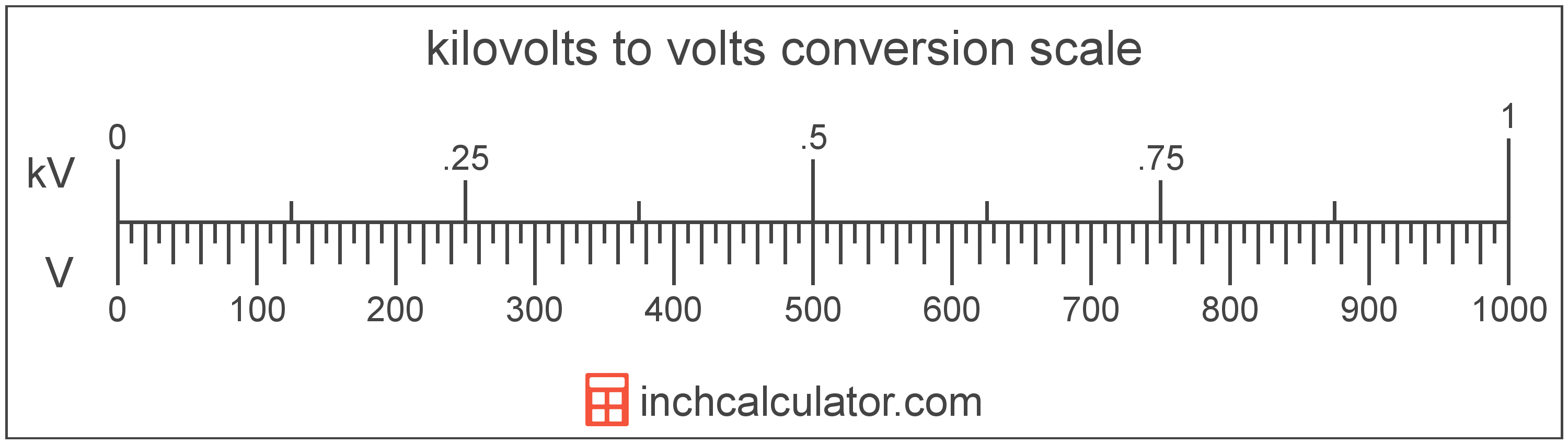# Volts to Kilovolts Converter

Enter the voltage in volts below to get the value converted to kilovolts.

Results in Kilovolts:1 V = 0.001 kV

Do you want to convert kilovolts to volts?

## How to Convert Volts to Kilovolts

To convert a measurement in volts to a measurement in kilovolts, divide the voltage by the following conversion ratio: 1,000 volts/kilovolt.

Since one kilovolt is equal to 1,000 volts, you can use this simple formula to convert:

kilovolts = volts ÷ 1,000

The voltage in kilovolts is equal to the voltage in volts divided by 1,000.

For example, here's how to convert 5,000 volts to kilovolts using the formula above.
kilovolts = (5,000 V ÷ 1,000) = 5 kV## What Is a Volt?

One volt is equal to the potential difference that would move one ampere of current against one ohm of resistance.

The volt is the SI derived unit for voltage in the metric system. Volts can be abbreviated as V; for example, 1 volt can be written as 1 V.

### Voltage and Ohm's Law

Voltage is a measurement of electromotive force and the electrical potential difference between two points of a conductor.

Ohm's Law states the current between two points on a conductor is proportional to the voltage and inversely proportional to the resistance. Using Ohm's Law, it's possible to express the potential difference in volts as an expression using current and resistance.

VV = IA × R

The potential difference in volts is equal to the current in amperes times the resistance in ohms.

## What Is a Kilovolt?

One kilovolt is equal to 1,000 volts, which are the potential difference that would move one ampere of current against one ohm of resistance.

The kilovolt is a multiple of the volt, which is the SI derived unit for voltage. In the metric system, "kilo" is the prefix for thousands, or 103. Kilovolts can be abbreviated as kV; for example, 1 kilovolt can be written as 1 kV.

## Volt to Kilovolt Conversion Table

Table showing various volt measurements converted to kilovolts.
Volts Kilovolts
1 V 0.001 kV
2 V 0.002 kV
3 V 0.003 kV
4 V 0.004 kV
5 V 0.005 kV
6 V 0.006 kV
7 V 0.007 kV
8 V 0.008 kV
9 V 0.009 kV
10 V 0.01 kV
20 V 0.02 kV
30 V 0.03 kV
40 V 0.04 kV
50 V 0.05 kV
60 V 0.06 kV
70 V 0.07 kV
80 V 0.08 kV
90 V 0.09 kV
100 V 0.1 kV
200 V 0.2 kV
300 V 0.3 kV
400 V 0.4 kV
500 V 0.5 kV
600 V 0.6 kV
700 V 0.7 kV
800 V 0.8 kV
900 V 0.9 kV
1,000 V 1 kV

## References

1. International Bureau of Weights and Measures, The International System of Units, 9th Edition, 2019, https://www.bipm.org/documents/20126/41483022/SI-Brochure-9-EN.pdf